Updating search results...

# 11 Results

View
Selected filters:
• OH.TMM.0006.Core.1Unrestricted Use
CC BY
Rating
0.0 stars

The Calculus II course was developed through the Ohio Department of Higher Education OER Innovation Grant. This work was completed and the course was posted in February&nbsp;2019.&nbsp;The course is part of the Ohio Transfer Module and is also named TMM006. For more information about credit transfer between Ohio colleges and universities, please visit: transfercredit.ohio.gov.Team LeadJim Fowler &nbsp; &nbsp; &nbsp; &nbsp; &nbsp; &nbsp; &nbsp; &nbsp; &nbsp; &nbsp;&nbsp;&nbsp;&nbsp;&nbsp;&nbsp;&nbsp;&nbsp;&nbsp;&nbsp;&nbsp;&nbsp;&nbsp;&nbsp;&nbsp;&nbsp;&nbsp;&nbsp;&nbsp;&nbsp;&nbsp; Ohio State UniversityRita Ralph&nbsp;&nbsp;&nbsp;&nbsp;&nbsp;&nbsp;&nbsp;&nbsp;&nbsp;&nbsp;&nbsp;&nbsp;&nbsp;&nbsp;&nbsp;&nbsp;&nbsp;&nbsp;&nbsp;&nbsp;&nbsp;&nbsp;&nbsp;&nbsp;&nbsp;&nbsp;&nbsp;&nbsp;&nbsp;&nbsp;&nbsp;&nbsp;&nbsp;&nbsp;&nbsp;&nbsp;&nbsp;&nbsp;&nbsp;&nbsp; Columbus State Community CollegeContent ContributorsNela Lakos &nbsp; &nbsp;&nbsp; &nbsp;&nbsp;&nbsp;&nbsp;&nbsp;&nbsp;&nbsp;&nbsp;&nbsp;&nbsp;&nbsp;&nbsp;&nbsp;&nbsp;&nbsp;&nbsp;&nbsp;&nbsp;&nbsp;&nbsp;&nbsp;&nbsp;&nbsp;&nbsp;&nbsp;&nbsp;&nbsp;&nbsp;&nbsp;&nbsp;&nbsp;&nbsp; Ohio State UniversityBart Snapp &nbsp; &nbsp; &nbsp; &nbsp; &nbsp; &nbsp; &nbsp;&nbsp; &nbsp;&nbsp;&nbsp;&nbsp;&nbsp;&nbsp;&nbsp;&nbsp;&nbsp;&nbsp;&nbsp;&nbsp;&nbsp;&nbsp;&nbsp;&nbsp;&nbsp;&nbsp;&nbsp;&nbsp;&nbsp;&nbsp; Ohio State UniversityJames Talamo&nbsp; &nbsp; &nbsp; &nbsp; &nbsp; &nbsp; &nbsp; &nbsp; &nbsp; &nbsp; &nbsp; &nbsp; &nbsp; &nbsp; &nbsp; &nbsp; &nbsp; Ohio State UniversityXiang Yan&nbsp;&nbsp;&nbsp;&nbsp;&nbsp;&nbsp;&nbsp;&nbsp;&nbsp;&nbsp;&nbsp;&nbsp;&nbsp;&nbsp;&nbsp;&nbsp;&nbsp;&nbsp;&nbsp;&nbsp;&nbsp;&nbsp;&nbsp;&nbsp;&nbsp;&nbsp;&nbsp;&nbsp;&nbsp;&nbsp;&nbsp;&nbsp;&nbsp;&nbsp;&nbsp;&nbsp;&nbsp;&nbsp;&nbsp;&nbsp; Edison State Community CollegeLibrarianDaniel Dotson&nbsp;&nbsp;&nbsp; &nbsp; &nbsp; &nbsp; &nbsp; &nbsp; &nbsp; &nbsp; &nbsp; &nbsp; &nbsp; &nbsp; &nbsp; &nbsp; &nbsp; &nbsp; &nbsp; Ohio State University&nbsp;&nbsp;&nbsp;&nbsp;&nbsp;&nbsp;&nbsp;&nbsp;&nbsp;&nbsp;&nbsp;&nbsp;&nbsp;&nbsp;&nbsp;&nbsp;&nbsp;&nbsp;&nbsp;&nbsp;&nbsp;Review TeamThomas Needham&nbsp;&nbsp;&nbsp;&nbsp;&nbsp;&nbsp;&nbsp;&nbsp;&nbsp;&nbsp;&nbsp;&nbsp;&nbsp;&nbsp;&nbsp;&nbsp;&nbsp;&nbsp;&nbsp;&nbsp;&nbsp;&nbsp;&nbsp;&nbsp;&nbsp;&nbsp;&nbsp;&nbsp; Ohio State UniversityCarl Stitz&nbsp;&nbsp;&nbsp;&nbsp;&nbsp;&nbsp;&nbsp;&nbsp;&nbsp;&nbsp;&nbsp;&nbsp;&nbsp;&nbsp;&nbsp;&nbsp;&nbsp;&nbsp;&nbsp;&nbsp;&nbsp;&nbsp;&nbsp;&nbsp;&nbsp;&nbsp;&nbsp;&nbsp;&nbsp;&nbsp;&nbsp;&nbsp;&nbsp;&nbsp;&nbsp;&nbsp;&nbsp;&nbsp;&nbsp;&nbsp;&nbsp;&nbsp;&nbsp; Lakeland Community CollegeSara Rollo &nbsp; &nbsp; &nbsp; &nbsp; &nbsp; &nbsp; &nbsp; &nbsp; &nbsp; &nbsp; &nbsp; &nbsp; &nbsp; &nbsp; &nbsp; &nbsp; &nbsp; &nbsp; &nbsp; &nbsp; North Central State College

Subject:
Calculus
Mathematics
Material Type:
Full Course
Provider:
Ohio Open Ed Collaborative
09/26/2018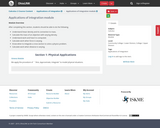Conditional Remix & Share Permitted
CC BY-NC
Rating
0.0 stars

After completing this section, students should be able to do the following.Understand linear density and its connection to mass.Calculate the mass of an objection with varying density.Understand work and how it is computed.Calculate work when force is varying.Know when to integrate a cross-section to solve a physics problem.Calculate work when distance is varying.

Subject:
Calculus
Material Type:
Module
07/25/2019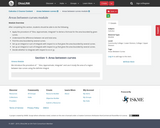Conditional Remix & Share Permitted
CC BY-NC
Rating
0.0 stars

After completing this section, students should be able to do the following.Apply the procedure of &ldquo;Slice, Approximate, Integrate&rdquo; to derive a formula for the area bounded by given curves.Understand the difference between net and total area.Find the area bounded by several curves.Set up an integral or sum of integrals with respect to&nbsp;xx&nbsp;that gives the area bounded by several curves.Set up an integral or sum of integrals with respect to&nbsp;yy&nbsp;that gives the area bounded by several curves.Decide whether to integrate with respect to&nbsp;xx&nbsp;or&nbsp;yy.

Subject:
Calculus
Material Type:
Module
07/24/2019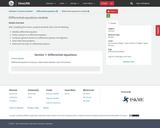Conditional Remix & Share Permitted
CC BY-NC
Rating
0.0 stars

After completing this section, students should be able to do the following.Identify a differential equation.Verify a solution to a differential equation.Compute a general solution to a differential equation via integration.Solve initial value problems.Determine the order of a differential equation.&nbsp;

Subject:
Calculus
Material Type:
Module
07/26/2019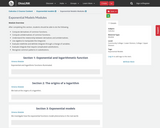Conditional Remix & Share Permitted
CC BY-NC
Rating
0.0 stars

After completing this section, students should be able to do the following.Compute derivatives of common functions.Compute antiderivatives of common functions.Understand the relationship between derivatives and antiderivatives.Use algebra to manipulate the integrand.Evaluate indefinite and definite integrals through a change of variables.Evaluate integrals that require complicated substitutions.Recognize common patterns in substitutions.

Subject:
Calculus
Material Type:
Module
07/25/2019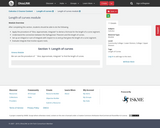Conditional Remix & Share Permitted
CC BY-NC
Rating
0.0 stars

After completing this section, students should be able to do the following.Apply the procedure of &ldquo;Slice, Approximate, Integrate&rdquo; to derive a formula for the length of a curve segment.Understand the connection between the Pythagorean Theorem and the length of curves.Set up an integral or sum of integrals with respect to&nbsp;xx&nbsp;and&nbsp;yy&nbsp;that gives the length of a curve segment.Evaluate integrals that involve square roots.

Subject:
Calculus
Material Type:
Module
07/25/2019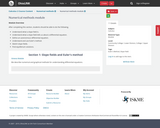Conditional Remix & Share Permitted
CC BY-NC
Rating
0.0 stars

After completing this section, students should be able to do the following.Understand what a slope field is.Understand what a slope field tells us about a differential equation.Define an autonomous differential equation.Understand and use Euler&rsquo;s method.Sketch slope fields.Find equilibrium solutions.

Subject:
Calculus
Material Type:
Module
07/26/2019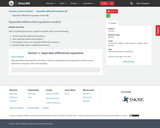Conditional Remix & Share Permitted
CC BY-NC
Rating
0.0 stars

After completing this section, students should be able to do the following.Identify separable differential equations.Solve separable differential equations.Find implicit solutions for separable differential equations.Identify steady-state (or equilibrium) solutions.

Subject:
Calculus
Material Type:
Module
07/26/2019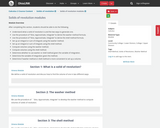Conditional Remix & Share Permitted
CC BY-NC
Rating
0.0 stars

After completing this section, students should be able to do the following.Understand what a solid of revolution is and the two ways to generate one.Use the procedure of &ldquo;Slice, Approximate, Integrate&rdquo; to derive the washer method formula.Use the procedure of &ldquo;Slice, Approximate, Integrate&rdquo; to derive the shell method formula.Set up an integral or sum of integrals using the washer method.Set up an integral or sum of integrals using the shell method.Compute volumes using the washer method.Compute volumes using the shell method.Determine whether to use washer or shell method given the variable of integration.Determine the variable of integration given the method.Determine if washer method or shell method is more convenient to set up a volume.

Subject:
Calculus
Material Type:
Module
07/25/2019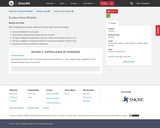Conditional Remix & Share Permitted
CC BY-NC
Rating
0.0 stars

After completing this section, students should be able to do the following.Know the definition of a frustum.Know how to compute the surface area of a frustum.Set-up an integral to compute the area of a surface of revolution in terms of&nbsp;xx.Set-up an integral to compute the area of a surface of revolution in terms of&nbsp;yy.Compute the area of a surface of revolution.

Subject:
Calculus
Material Type:
Module
07/25/2019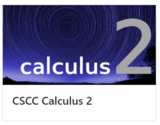Unrestricted Use
CC BY
Rating
0.0 stars

This is a Calculus II interactive textbook with modules curated and created on The Ohio State University's Ximera platform.

Subject:
Calculus
Mathematics
Material Type:
Textbook
Provider:
Columbus State Community College
Author:
Kevin James
Lee Wayand
The Ximera Project
Tzy-Yi "Alan" Yang